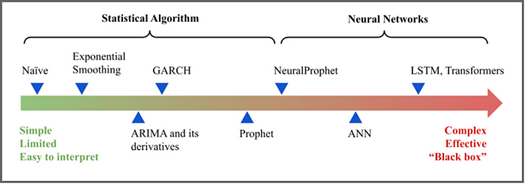# 机器学习算法之K-近邻（KNN）

## 什么是K-近邻算法？

K近邻法(k-nearest neighbor, k-NN)是1967年由Cover T和Hart P提出的一种基本分类与回归方法。它的工作原理是：存在一个样本数据集合，也称作为训练样本集，并且样本集中每个数据都存在标签，即我们知道样本集中每一个数据与所属分类的对应关系。输入没有标签的新数据后，将新的数据的每个特征与样本集中数据对应的特征进行比较，然后算法提取样本最相似数据(最近邻)的分类标签。一般来说，我们只选择样本数据集中前k个最相似的数据，这就是k-近邻算法中k的出处，通常k是不大于20的整数。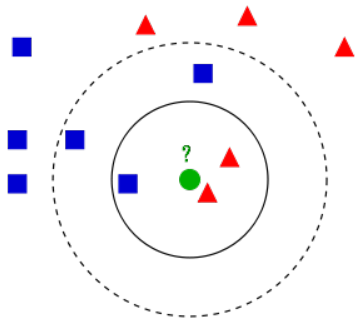k近邻算法例子。测试样本（绿色圆形）应归入要么是第一类的蓝色方形或是第二类的红色三角形。如果k=3（实线圆圈）它被分配给第二类，因为有2个三角形和只有1个正方形在内侧圆圈之内。如果k=5（虚线圆圈）它被分配到第一类（3个正方形与2个三角形在外侧圆圈之内）。

• 当选择的K值较小时，用于预测的训练数据集则较小，此时近似误差会减小，但是其估计误差会增大，预测结果对近邻的实例点非常敏感。若此时近邻的实例点是噪声，预测就会出错。
• 当选择的K值较大时，用于预测的训练数据集则较大，此时估计误差会减小，但是其近似误差又会增大，此时与输入实例较远(不相似)的实例也会对预测起作用，使预测发生错误。

KNN算法的优缺点

• 优点：精度高、对异常值不敏感、无数据输入假定
• 缺点：计算复杂度高、空间复杂度高（在高维情况下，会遇到『维数灾难』的问题）

## KNN算法三要素

KNN算法我们主要考虑三个重要的要素，对于固定的训练集，只要这三点确定了，算法的预测方式也就决定了。这三个最终的要素距离度量、 k值的选择和分类决策规则决定。

### K值的选择

k值的大小决定了邻域的大小。较小的k值使得预测结果对近邻的点非常敏感，如果近邻的点恰好是噪声，则预测便会出错。话句话说，k值的减小意味着整体模型变得复杂，容易发生过拟合。较大的k值会让输入实例中较远的（不相似的）训练实例对预测起作用，使预测发生错误，k值的增大意味着整体模型变得简单。在实际的应用中，一般采用一个比较小的K值。并采用交叉验证的方法，选取一个最优的K值。一个极端是k等于样本数m，则完全没有分类，此时无论输入实例是什么，都只是简单的预测它属于在训练实例中最多的类，模型过于简单。

### 分类决策规则决定

k近邻法中的分类规则往往是多数表决，即由输入实例的k个近邻的训练实例中的多数类决定输入的实例。但这个规则存在一个潜在的问题：有可能多个类别的投票数同为最高。这个时候，可以通过以下几个途径解决该问题：

• 从投票数相同的最高类别中随机地选择一个；
• 通过距离来进一步给票数加权；
• 减少K的个数，直到找到一个唯一的最高票数标签。

## K-近邻算法的实现

### 线性扫描

KNN的最简单朴素的方法即直接线性扫描，大致步骤如下：

• 计算待预测数据与各训练样本之间的距离
• 按照距离递增排序
• 选择距离最小的k个点
• 计算这k个点类别的频率，最高的即为待预测数据的类别。
import math
from collections import defaultdict

class KNN(object):
def __init__(self, k=2):
self.data = None
self.k = k

@staticmethod
def distance(p1, p2):
x1, y1 = p1
x2, y2 = p2

x_ = x2 - x1
y_ = y2 - y1

return math.sqrt(x_ ** 2 + y_ ** 2)

def fit(self, X, y):
self.data = dict(zip(X, y))

def predict(self, point):
distances = {}

for p, _ in self.data.items():
distances[p] = self.distance(p, point)

sort_distances = dict(sorted(distances.items(), key=lambda x: x))
topk = defaultdict(int)
for idx, (p, v) in enumerate(sort_distances.items()):
if idx == self.k - 1:
break
topk[self.data[p]] += 1

topk = sorted(topk.items(), key=lambda x: -x)

if __name__ == '__main__':
points = [(1, 1), (1, 1.2), (0, 0), (0, 0.2), (3, 0.5), (3.3, 0.9)]
labels = ['A', 'A', 'B', 'B', 'C', 'C']

knn = KNN()
knn.fit(points, labels)
label = knn.predict((0.9, 0.7))
print(label)


### KD树

KD树(k-dimensional tree)，也可称之为K维树，可以用更高的效率来对空间进行划分，并且其结构非常适合寻找最近邻居和碰撞检测。对于2维空间，KD树可称为2D树，因为空间只有两个坐标轴；对于3维空间，KD树可称为3D树，空间中有三个坐标轴；以此类推。

• 选定一个轴，比如X轴，选择这个轴上的中位数的所在点为根节点
• 所有X比中位数X小的，都划分为左子树；反之，则划分为右子树
• 对于左右两个子树，重复第一步，但是需要把划分轴换成另外一个轴（Y）继续
• 重复以上过程，直到所有点都加入KD树中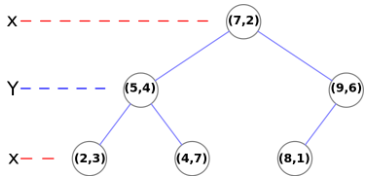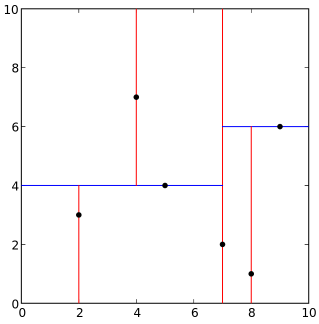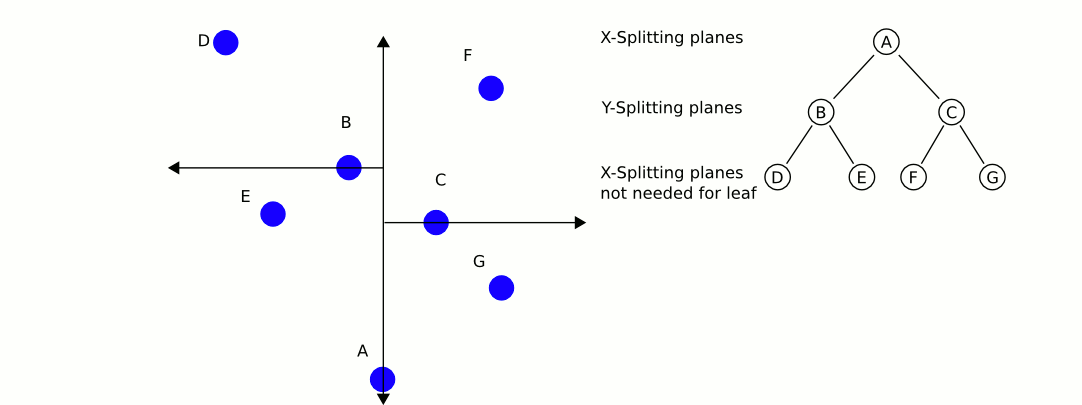1. 建立一个空的栈S
2. 对于给定的查询点P，沿着根节点遍历整个KD树，直到不能再遍历为止，将每个遍历的点都入栈（Push）
3. 遍历的过程非常简单，对于KD树中的点和这个点的划分坐标，如果查询点比这个点的划分坐标大，则继续遍历这个点的右子树，否则遍历这个点的左子树
4. 若栈非空，开始循环，设最邻近距离为无穷大
5. 将栈顶的点P弹出（Pop），计算查询点与之的距离Dist，如果Dist小于最邻近距离，则更新最近邻距离为Dist，同时更新最邻近点为P
6. 判断点P的划分轴，若查询点到划分轴的距离小于最近邻距离，则说明在划分轴的另外一侧还可能存在更邻近的点，需要在划分轴的另一侧的根节点再执行一次遍历，将每个遍历的点都入栈（Push）
7. 若栈为空，则终止循环，返回结果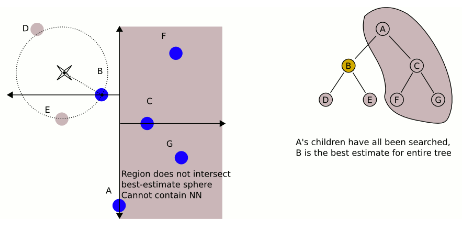KD树之所以如此高效的原因在于第六步，也就是剪枝。如上图所示，在已经搜索到B时，发现其到B的距离，要比到A的右子树的平面距离还更短，所以整个A的右子树都被剪枝，一下子剪去了一半的点。

# -*- coding: utf-8 -*-
import math
from collections import defaultdict

class KNN(object):
def __init__(self, topk=3):
self.data = None
self.store = {}
self.topk = topk

def build_kdtree(self, points, depth=0):
n = len(points)
if n <= 0:  # 如果当前子空间已经没有点了则构建过程结束
return None

axis = depth % 2  # 计算当前选择的坐标轴
sorted_points = sorted(points, key=lambda point: point[axis])  # 对当前子空间的点根据当前选择轴的值进行排序
median = n // 2  # 中位数取排序后坐标点的中间位置的数

return {
'point': sorted_points[median],  # 当前根结点
'left': self.build_kdtree(sorted_points[:median], depth + 1),  # 将超平面左边的点交由左子结点递归操作
'right': self.build_kdtree(sorted_points[median + 1:], depth + 1)  # 同理，将超平面右边的点交由右子结点递归操作
}

@staticmethod
def distance(p1, p2):
if p1 is None or p2 is None:
return 0

x1, y1 = p1
x2, y2 = p2

x_ = x2 - x1
y_ = y2 - y1

return math.sqrt(x_ ** 2 + y_ ** 2)

def closer_distance(self, point, p1, p2):

d1 = self.distance(point, p1)
d2 = self.distance(point, p2)

if p1 is None:
return (p2, d2)
if p2 is None:
return (p1, d1)

return (p1, d1) if d1 < d2 else (p2, d2)

def kdtree_closest_point(self, root, point, depth=0):
if root is None:
return None

axis = depth % 2

next_branch = None
opposite_branch = None

# 以下主要是比较当前点到根结点和两个子结点之间的距离
if point[axis] < root['point'][axis]:
next_branch = root['left']
opposite_branch = root['right']
else:
next_branch = root['right']
opposite_branch = root['left']

best, closer_dist = self.closer_distance(
point,
self.kdtree_closest_point(
next_branch,
point,
depth + 1),
root['point']
)

if self.distance(point, best) > abs(point[axis] - root['point'][axis]):
best, closer_dist = self.closer_distance(
point,
self.kdtree_closest_point(
opposite_branch,
point,
depth + 1),
best
)

# 储存距离，留作投票用
if best in self.store and self.store[best] > closer_dist:
self.store[best] = closer_dist
else:
self.store[best] = closer_dist

return best

def fit(self, X, y):
self.data = dict(zip(X, y))
self.kdtree = self.build_kdtree(X)

def predict(self, point):
# best 是最邻近的点
best = self.kdtree_closest_point(self.kdtree, point)

sorted_stores = sorted(self.store.items(), key=lambda x: x)[:self.topk]
counter = defaultdict(int)
for candidates, score in sorted_stores:
counter[self.data[candidates]] += 1

# 按照投票数降序排列
sorted_counter = sorted(counter.items(), key=lambda x: -x)
counter = list(counter.items())

if len(counter) > 1:
if counter != counter:
best = counter

return self.data[best]

if __name__ == '__main__':
points = [(1, 1), (1, 1.2), (0, 0), (0, 0.2), (3, 0.5), (3.3, 0.9)]
labels = ['A', 'A', 'B', 'B', 'C', 'C']

knn = KNN(topk=3)
knn.fit(points, labels)
label = knn.predict((0.9, 0.9))
print(label)

## 使用Scikit-Learn进行分类预测

定义数据：
# 定义特征变量
weather = ['Sunny','Sunny','Overcast','Rainy','Rainy','Rainy','Overcast','Sunny','Sunny',
'Rainy','Sunny','Overcast','Overcast','Rainy']
temperature = ['Hot','Hot','Hot','Mild','Cool','Cool','Cool','Mild','Cool','Mild','Mild','Mild','Hot','Mild']

# 定义分类标签
play=['No','No','Yes','Yes','Yes','No','Yes','No','Yes','Yes','Yes','Yes','Yes','No']

# 将特征变量和分类标签数值化
from sklearn import preprocessing

le = preprocessing.LabelEncoder()
weather_encoded=le.fit_transform(weather)
temperature_encoded=le.fit_transform(temperature)

# 整合特征变量
features=list(zip(weather_encoded,temperature_encoded))

label=le.fit_transform(play)

from sklearn.neighbors import KNeighborsClassifier

model = KNeighborsClassifier(n_neighbors=3)
model.fit(features,label)
predicted= model.predict([[0,2]]) # 0:Overcast, 2:Mild
print(predicted)


## 使用Grid Search确定K值

from sklearn import datasets

print(wine.feature_names) #获取特性向量名称
print(wine.target_names) #获取分类标签名称

print(wine.data[0:5]) #查看特征向量数据
print(wine.target) #查看分类标签数据
print(wine.data.shape)
print(wine.target.shape)

from sklearn.model_selection import train_test_split

# 将数据划分为训练集和测试集
X_train, X_test, y_train, y_test = train_test_split(wine.data, wine.target, test_size=0.3) # 70% training and 30% test

import numpy as np
from sklearn.neighbors import KNeighborsClassifier

neighbors = np.arange(1,10)
train_accuracy =np.empty(len(neighbors))
test_accuracy = np.empty(len(neighbors))

for i,k in enumerate(neighbors):
knn = KNeighborsClassifier(n_neighbors=k)
knn.fit(X_train, y_train)
train_accuracy[i] = knn.score(X_train, y_train) #使用训练集测试准确率
test_accuracy[i] = knn.score(X_test, y_test) #使用测试集测试准确率

import matplotlib.pyplot as plt
%matplotlib inline

plt.title('k-NN Varying number of neighbors')
plt.plot(neighbors, test_accuracy, label='Testing Accuracy')
plt.plot(neighbors, train_accuracy, label='Training accuracy')
plt.legend()
plt.xlabel('Number of neighbors')
plt.ylabel('Accuracy')
plt.show()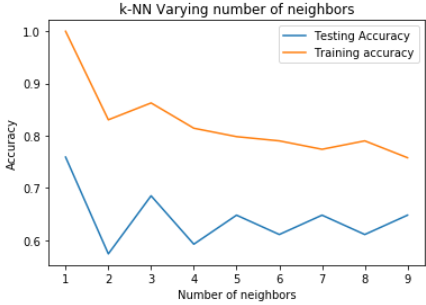## KNN分类实战演练

import numpy as np
import pandas as pd
import matplotlib.pyplot as plt

print(df.shape)
X = df.drop('Outcome',axis=1).values
y = df['Outcome'].values

from sklearn.model_selection import train_test_split
X_train,X_test,y_train,y_test = train_test_split(X,y,test_size=0.4,random_state=42, stratify=y)

from sklearn.neighbors import KNeighborsClassifier

neighbors = np.arange(1,9)
train_accuracy =np.empty(len(neighbors))
test_accuracy = np.empty(len(neighbors))

for i,k in enumerate(neighbors):
#Setup a knn classifier with k neighbors
knn = KNeighborsClassifier(n_neighbors=k)

#Fit the model
knn.fit(X_train, y_train)

#Compute accuracy on the training set
train_accuracy[i] = knn.score(X_train, y_train)

#Compute accuracy on the test set
test_accuracy[i] = knn.score(X_test, y_test)

plt.title('k-NN Varying number of neighbors')
plt.plot(neighbors, test_accuracy, label='Testing Accuracy')
plt.plot(neighbors, train_accuracy, label='Training accuracy')
plt.legend()
plt.xlabel('Number of neighbors')
plt.ylabel('Accuracy')
plt.show()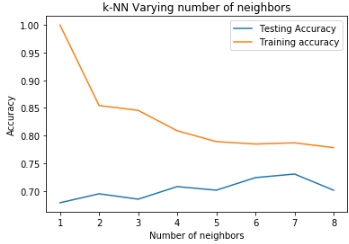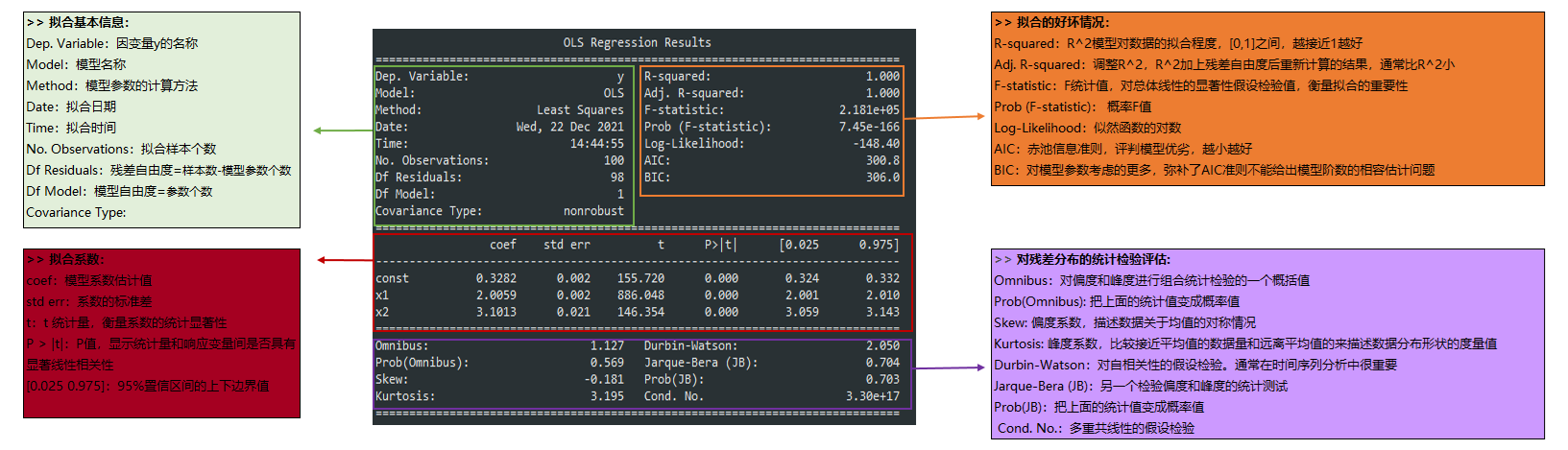##### Statsmodels 统计包之OLS回归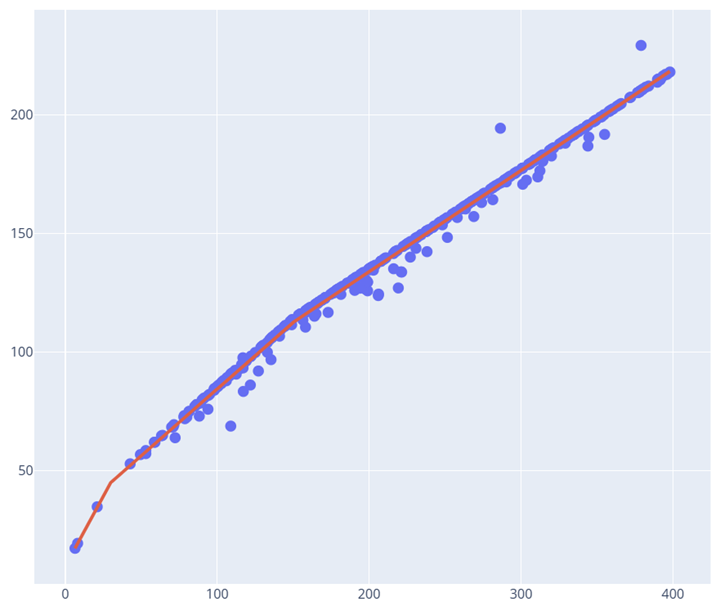##### 机器学习之分段线性回归